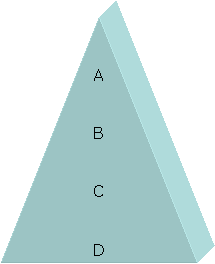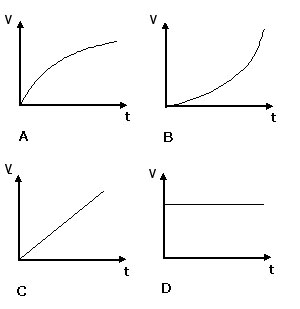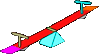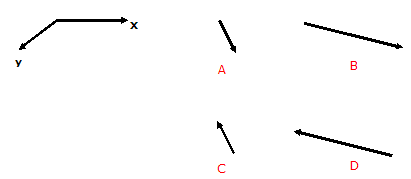CIE iGCSE - Triple Extras

Page 1

1. Which of these letters correctly shows the centre of gravity of this shape?2. Which of these graphs shows inceasing acceleration?3. If a force acts at 90 degrees to a moving vehicle, it will make the vehicle

• A). speed up
• B). slow down
• C). move in a curved path
• D). stop

4. If there are no resultant forces acting on a system, it is said to be in

• A). equilibrium
• B). balance
• C). stasis
• D). stability

These questions are about a see-saw. When different children sit on the see-saw they find they have to move to different points to make the see-saw balance. What are the missing values needed to make the see saw balance?

 Left Hand Side Right Hand Side Name mass (kg) distance from pivot (m) Name mass (kg) distance from pivot (m) 5. Abby 60 1.5 Charlie 90 ? 1 1.5 2 135 6. Borat 75 ? 0.83 1 1.2 1.5 Elin 45 2 7. Doris ? 79.8 40.7 167.6 38.0 2.1 Fred 57 1.4 8. ? Abby Borat Charlie Doris Elin Fred Goh ? 1.6 Goh 40 2.49. Which of the following are vector quantities?

• A). mass and velocity
• B). acceleration and force
• C). mass and displacement
• D). velocity and time

10.  Two vectors X and Y are to be added. Which of these correctly shows the result?When divers go deep under water, the pressure increases. What are the missing values in this table showing pressure increase ?

(Density = 1000 kg/m3)

 Pressure increase Depth 11. 400 000 Pa ? 4m 40m 400m 4000m 12. 650 kPa ? 6.5m 65m 650m 6500m 13. ? 250Pa 2500Pa 25000Pa 250000Pa 250cm

14. There are several devices used to measure pressure, including...

• A). manometers and barometers
• B). odometers and manometers
• C). odometers and barometers
• D). barometers and decimeters

15. What is 'Brownian Motion'?

• A) The vibrating of atoms
• B) the collision of particles in a gas
• C) The movement of substances across a pressure gradient
• D) The random motion of large smoke or pollen particles

16. It is difficult to measure very high temperatures with a standard thermometer. Instead, a device using a pair of metals in contact is used, called a

• A) manometer
• B) thermocouple
• C) thermistor
• D) fixed point device

17. A kettle is filled with 1 kg of boiling water. It is turned on and the energy supplied is measured as the water is boiled away. The mass of water remaining can be measured after a certain amount of energy is supplied.

This experiment is used to find the

• A) latent heat of vaporisation
• B) specific heat capacity of water
• C) fixed point for water
• D) fixed point for fusion